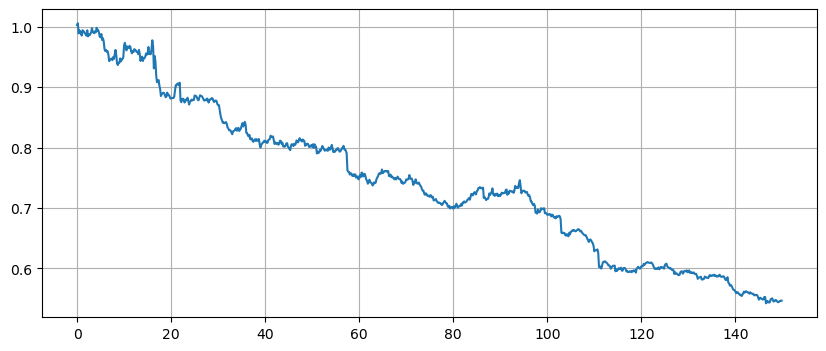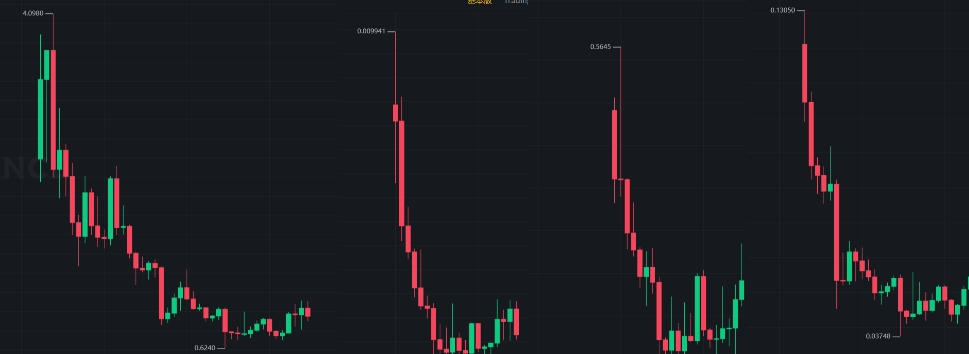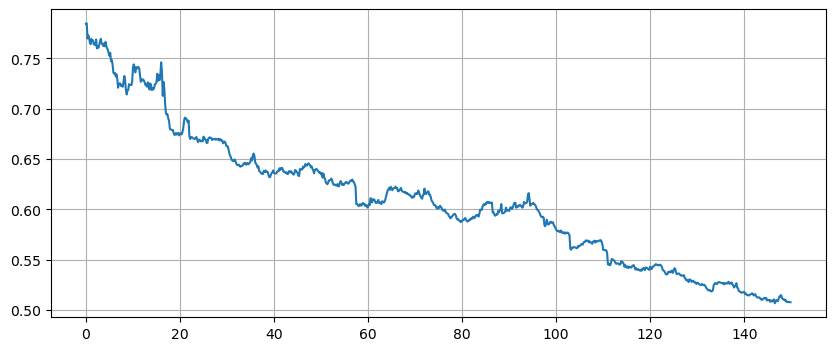# 币种上线永续合约后的价格表现

Author: 小草, Created: 2023-11-17 15:43:19, Updated: 2023-11-17 21:36:21### 数据准备

``````df = df_close/df_close.fillna(method='bfill').iloc
price_changes = {}
for coin in df.columns[df.iloc.isna()]:
listing_time = df[coin].first_valid_index()
price_changes[coin] = df[coin][df.index>listing_time].values
changes_df = pd.DataFrame.from_dict(price_changes, orient='index').T
changes_df.index = changes_df.index/6
changes_df = changes_df[changes_df.index<150]
changes_df.mean(axis=1).plot(figsize=(15,6),grid=True);
``````

### 结果分析### 排除指数影响

``````total_index = df.mean(axis=1)
df = df.divide(total_index,axis=0)
``````More